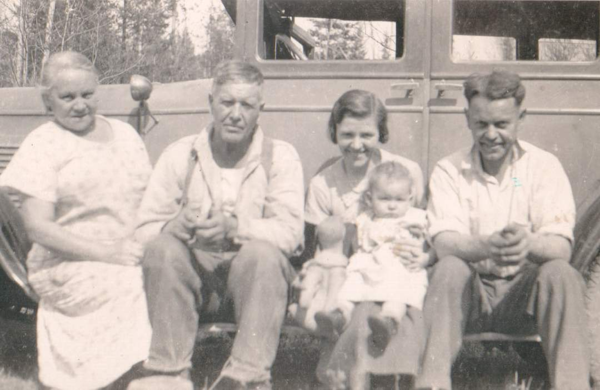## Out & About

Place mouse over face for identification of personDescription Out & About Date / Time 1936-01-20 Country / State / County / City / Placename CAN, BC Persons (t-b,l-r) Annie Clark McBride, Thomas James McBride, Martha Reichert McBride, Winnifred Anne McBride, Earl Thomas McBride
```lib card:
dc:
title 		=	Out & About
creator 	=	Jerome Goulet
subject 	=
description 	=	Original (scanned analog or digital), names to faces, stored to LibCard "format", within image exif:
publisher 	=	Jerome Goulet
contributor 	=
date 		=	1936-01-20
resourcetype 	=
iformat 	=
if_mime 	=	image/png
if_resolution 	=	x
if_dimensions 	=
if_dim_x 	=	600
if_dim_y 	=	390
resourceID 	=
source 		=	photograph (analog or digital)
language 	=	eng
rights 		=	Copyright 2020, Jerome Goulet<jtgoulet@yahoo.com>, GNU General Public License
lc:
when:
dateUTC		=	1936-01-20
timeUTC		=	0000
tz		=	+0000

dateLocal	=	1936-01-20
timeLocal	=	0000
where:
admin0		=	CAN
admin1		=	BC
admin2		=	x
admin3		=	x
pName		=	x
lat		=	x
lon		=	x
who:
person:
name:
surName		=	McBride
givenName	=	Annie Clark
aka		=	ACM
relation	=
pip:	(person in picture)
boxX		=	49
boxY		=	73
boxWidth	=	59
boxHeight	=	59
label		=
uri		=
person:
name:
surName		=	McBride
givenName	=	Thomas James
aka		=	TJM
relation	=
pip:	(person in picture)
boxX		=	207
boxY		=	82
boxWidth	=	59
boxHeight	=	59
label		=
uri		=
person:
name:
surName		=	McBride
givenName	=	Martha Reichert
aka		=	MRM
relation	=
pip:	(person in picture)
boxX		=	329
boxY		=	114
boxWidth	=	56
boxHeight	=	56
label		=
uri		=
person:
name:
surName		=	McBride
givenName	=	Winnifred Anne
aka		=	WAM
relation	=
pip:	(person in picture)
boxX		=	374
boxY		=	178
boxWidth	=	50
boxHeight	=	50
label		=
uri		=
person:
name:
surName		=	McBride
givenName	=	Earl Thomas
aka		=	ETM
relation	=
pip:	(person in picture)
boxX		=	461
boxY		=	101
boxWidth	=	61
boxHeight	=	61
label		=
uri		=
what:
eventName	=	Out & About
```